## 15.2 Enumerating cases

The simplest type of simulation merely explicates information that is fully contained in a problem description. This promotes insight by showing the consequences of premises.

### 15.2.1 Bayesian situations

For several decades, the psychology of reasoning and problem solving has been haunted by a class of problems. The drosophila task of this field is the so-called mammography problem (based on Eddy, 1982).

#### The mammography problem

The following version of the mammography problem stems from (using the standard probability format):

1. The probability of breast cancer is 1% for a woman at age forty who participates in routine screening.

2. If a woman has breast cancer, the probability is 80% that she will get a positive mammography.

3. If a woman does not have breast cancer, the probability is 9.6% that she will also get a positive mammography.

A woman in this age group had a positive mammography in a routine screening.
What is the probability that she actually has breast cancer?

Do not despair when you find this problem difficult. Only about 4% of naive people successfully solve this problem .

### 15.2.2 Analysis

We should first identify precisely what is given and asked in this problem:

#### What is given?

Identifying the information provided by the problem:

1. Prevalence of the condition (cancer) $$C$$: $$p(C)=.01$$

2. Sensitivity of the diagnostic test $$T$$: $$p(T|C)=.80$$

3. False positive rate of the diagnostic test $$T$$: $$p(T|¬C)=.096$$

Identifying the question: What would solve the problem?

• The conditional probability of the condition, given a positive test result. This is known as the test’s positive predictive value (PPV): $$p(C|T)=?$$.

#### Bayesian solution

The problem provides an unconditional ($$p(C)$$) and two conditional probabilities ($$p(T|C)$$ and $$p(T|¬C)$$) and asks for the inverse of one conditional probability (i.e., $$p(C|T)$$). Problems of this type are often framed as requiring Bayesian reasoning’’, as their mathematical solution can be derived by a formula known as Bayes’ theorem:

$p(C|T) = \frac{p(C) \cdot p(T|C) } {p(C) \cdot p(T|C)~+~p(\neg C) \cdot p(T|\neg C) } = \frac{.01 \cdot .80 } { .01 \cdot .80 ~+~ (1 - .01) \cdot .096 } \approx\ 7.8\%.$

This looks (and is) pretty complicated — and it’s not surprising that hardly anyone solves the problem correctly.

Fortunately, there are much simpler ways of solving this and related problems. Perhaps the most well-known account of so-called “facilitation effects” is provided by . The following simulation essentially implements a version of the problem that expresses the probabilities provided by the problem in terms of natural frequencies for a population of $$N$$ individuals. This will allow us to replace complex probabilistic calculations by simpler enumerations of cases.

### 15.2.3 Solution by enumeration

A first approach assumes a population of N individuals and applies the given probabilities to them. We can then inspect the population to derive the desired result.

All fixed, no sampling:

# Parameters:
N <- 1000     # population size
prev <- .01   # condition prevalence
sens <- .80   # sensitivity of the test
fart <- .096  # false alarm rate of the test (i.e., 1 - specificity)

Prepare data structures: Two vectors of length N:

# Prepare data structures:
cond <- rep(NA, N)
test <- rep(NA, N)

Using the information given to compute the expected values (i.e., the number of corresponding individuals if those probabilities are perfectly accurate):

# Compute expected values:
n_cond <- round(prev * N, 0)
n_cond_test <- round(sens * n_cond, 0)
n_false_pos <- round(fart * (N - n_cond), 0)

Note that we needed to make two non-trivial decisions in computing the expected values:

• Level of accuracy: Rounding to nearest integers
• Priority: Using value of n_cond in other computations ensures consistency (but also dependencies)

Using these numbers to categorize a corresponding number of elements of the cond and test vectors:

# Applying numbers to population (by subsetting):

# (a) condition:
cond[1:N] <- "healthy"
if (n_cond >= 1){ cond[1:n_cond] <- "cancer" }
cond <- factor(cond, levels = c("cancer", "healthy"))

# (b) test by condition == "cancer":
test[cond == "cancer"] <- "negative"
if (n_cond_test >= 1) {test[cond == "cancer"][1:n_cond_test] <- "positive"}

# (c) test by condition == "healthy":
test[cond == "healthy"] <- "negative"
if (n_false_pos >= 1) {test[cond == "healthy"][1:n_false_pos] <- "positive"}
test <- factor(test, levels = c("positive", "negative"))

Analogies:

The following visualizations use the riskyr package :

• Drawing a tree of natural frequencies: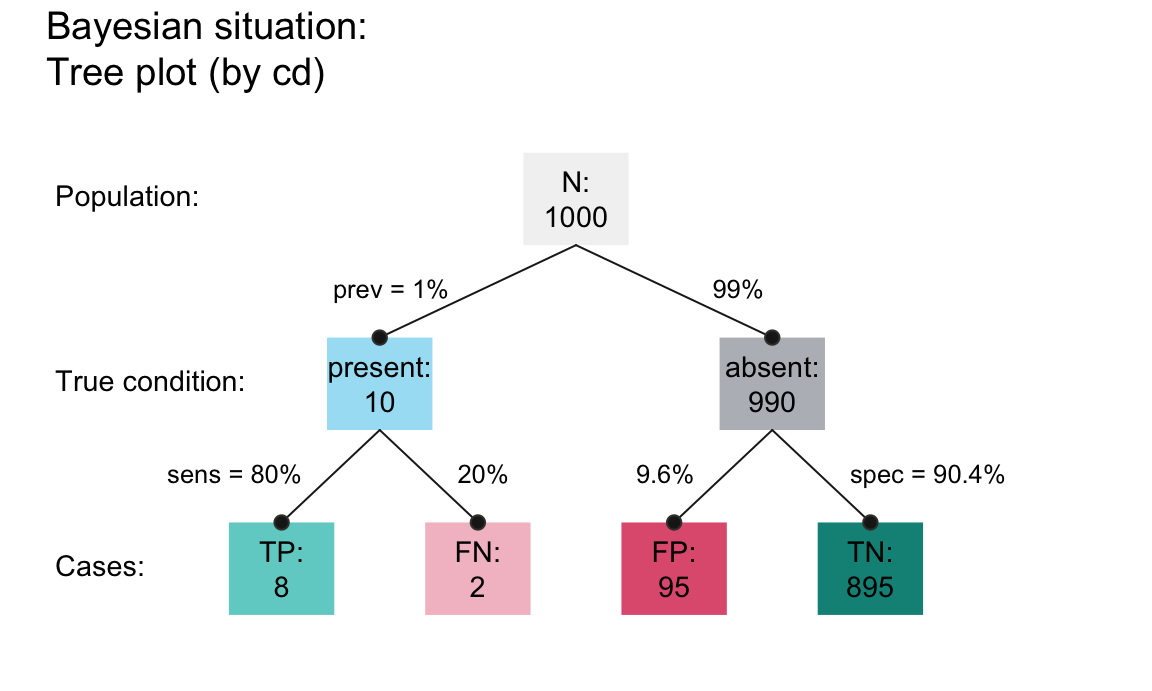Visualizing the individual items of the population:

• Coloring the N squares of an icon array in three steps: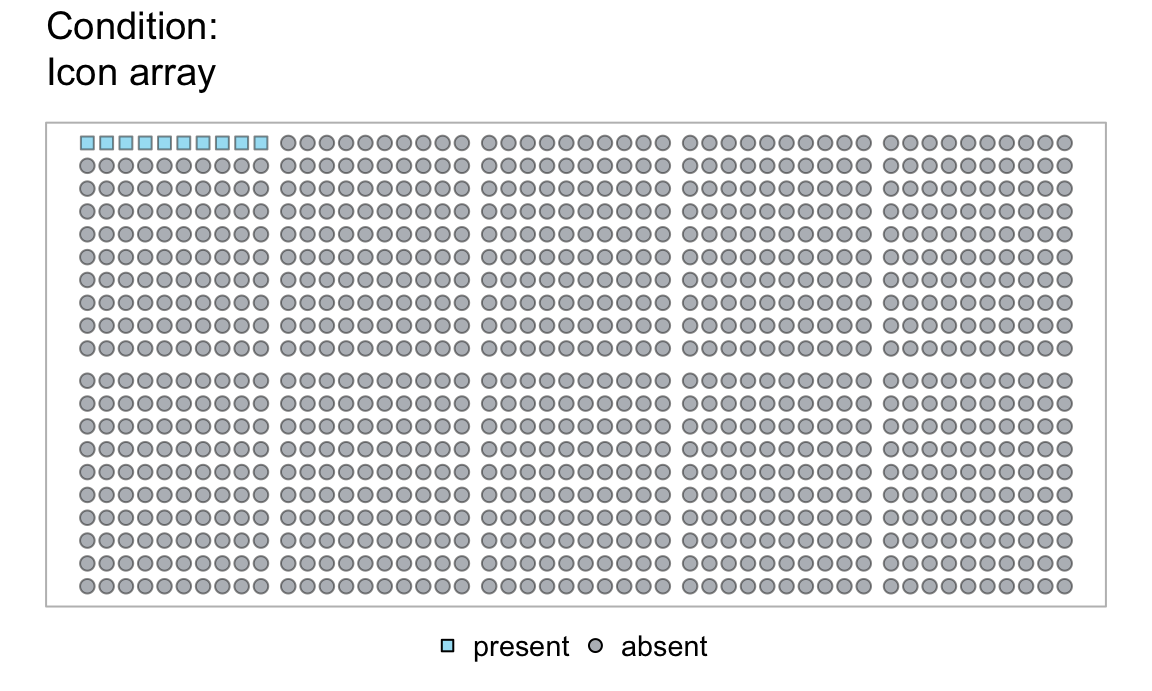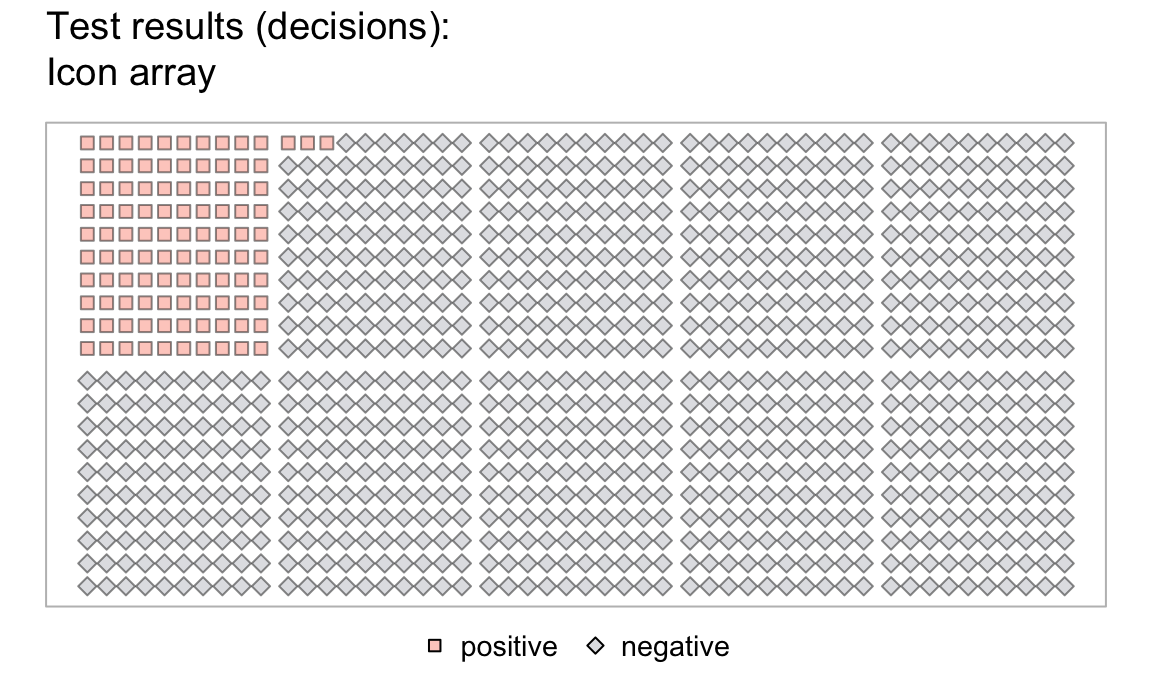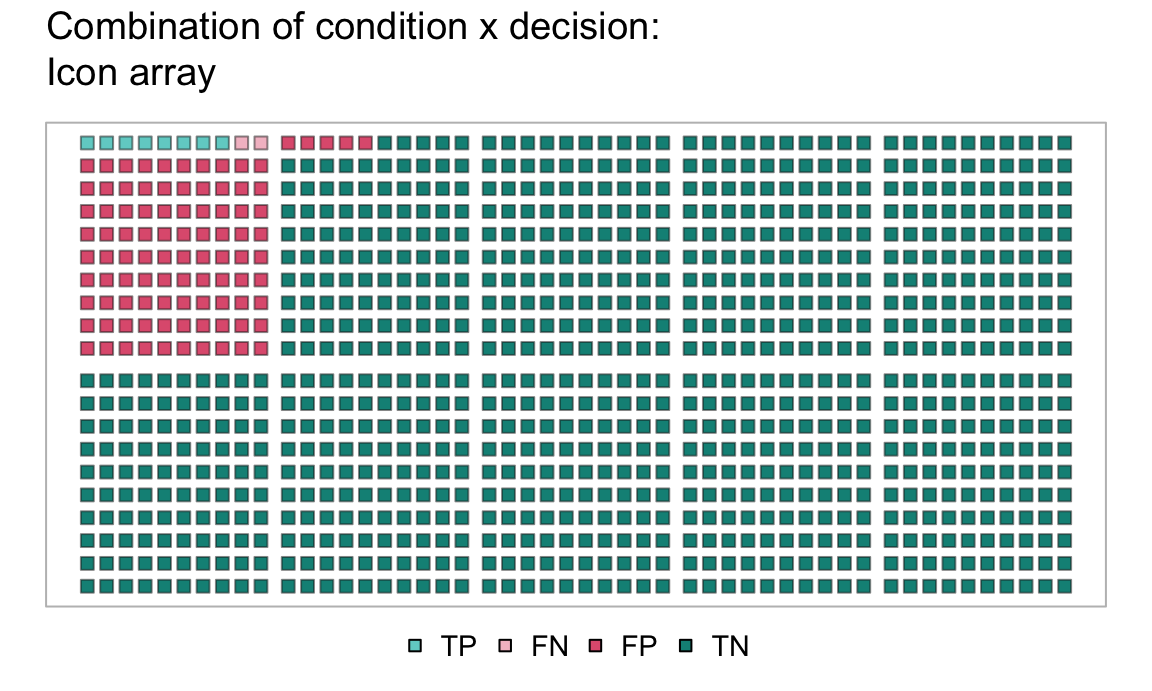Our result are two vectors (cond and test) with binary categories, which now can be inspected.

Conduct some checks:

# Checks: Are the categories exhaustive?
sum(cond == "cancer")   + sum(cond == "healthy")  == N
#>  TRUE
sum(test == "positive") + sum(test == "negative") == N
#>  TRUE

# Crosstabulation:
table(test, cond)
#>           cond
#> test       cancer healthy
#>   positive      8      95
#>   negative      2     895

Answer by inspecting table (or the combination of vectors):

sum(test == "positive" & cond == "cancer")  # frequency of target individuals
#>  8
sum(test == "positive" & cond == "cancer") / sum(test == "positive")  # desired conditional probability
#>  0.0776699

Note that this solution used the probabilistic information, but required no random or statistical processes. Instead, we assumed a large population of N individuals and used the probabilities to enumerate the corresponding number (or proportions) of individuals in subgroups of the population. The resulting proportion of individuals who both test positive and suffer from cancer out of those with a positive test result matches our analytic solution of $$p(C|T) = 7.8\%$$ from above.

An alternative way of solving this problem could take probability more seriously by adding random sampling. We will leave this to Exercise 15.5.2, but use a different problem to illustrate sampling-based simulations (in Section 15.3).

### 15.2.4 Visualizing Bayesian situations

The following visualizations further illustrate the solutions for the Bayesian situation of the mammography problem that was derived in this section:

• A frequency net provides an overview of the subsets of frequencies (as nodes) and the probabilities (as edges between nodes):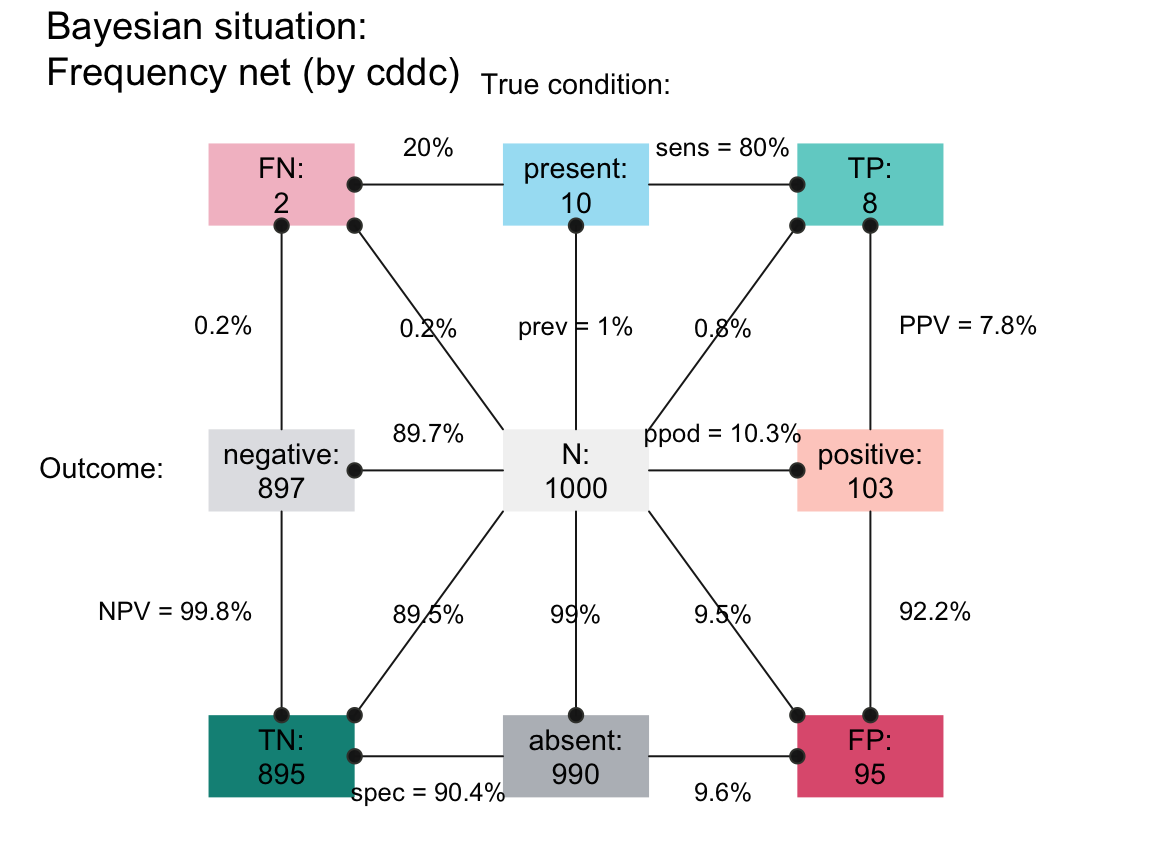• The same information can be expressed in two distinct frequency tree diagrams: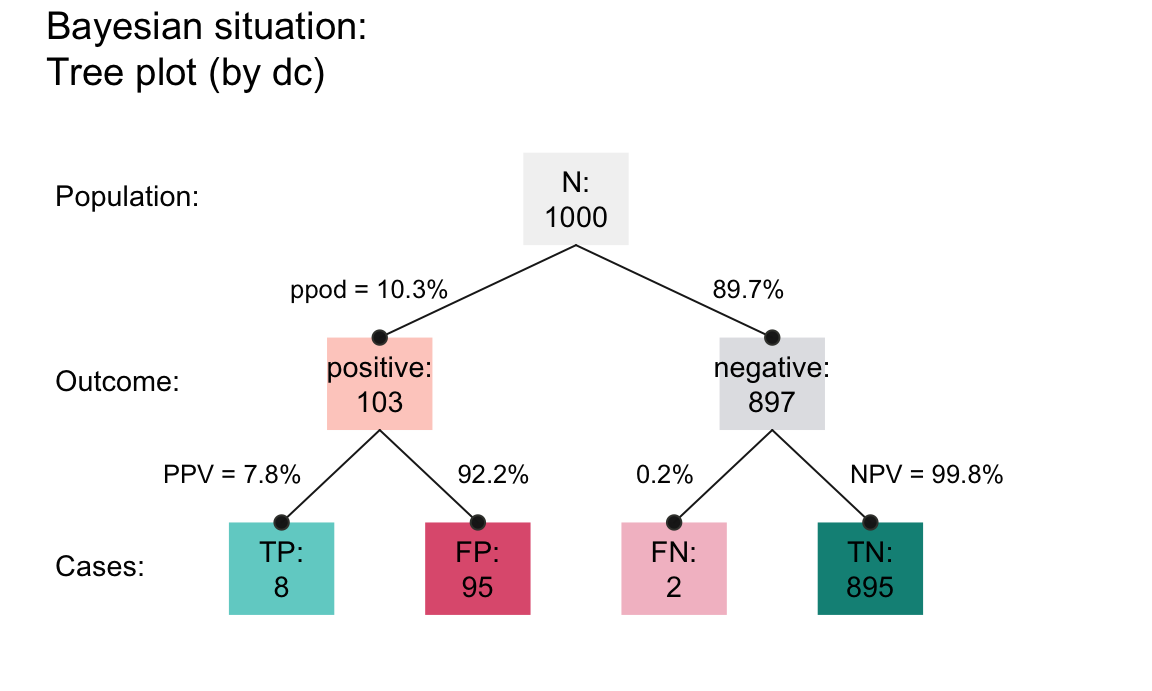The visualizations shown here use the riskyr package . See for the theoretical background of such problems.

### References

Binder, K., Krauss, S., & Wiesner, P. (2020). A new visualization for probabilistic situations containing two binary events: The frequency net. Frontiers in Psychology, 11, 750. https://doi.org/10.3389/fpsyg.2020.00750
Eddy, D. M. (1982). Probabilistic reasoning in clinical medicine: Problems and opportunities. In D. Kahneman, P. Slovic, & A. Tversky (Eds.), Judgment under uncertainty (pp. 249–267). Cambridge University Press. https://doi.org/10.1017/CBO9780511809477.019
Gigerenzer, G., & Hoffrage, U. (1995). How to improve Bayesian reasoning without instruction: Frequency formats. Psychological Review, 102(4), 684–704. https://doi.org/10.1037/0033-295X.102.4.684
McDowell, M., & Jacobs, P. (2017). Meta-analysis of the effect of natural frequencies on Bayesian reasoning. Psychological Bulletin, 143(12), 1273–1312. https://doi.org/10.1037/bul0000126
Neth, H., Gaisbauer, F., Gradwohl, N., & Gaissmaier, W. (2021). riskyr: Rendering risk literacy more transparent. https://riskyr.org/, https://CRAN.R-project.org/package=riskyr
Neth, H., Gradwohl, N., Streeb, D., Keim, D. A., & Gaissmaier, W. (2021). Perspectives on the 2x2 matrix: Solving semantically distinct problems based on a shared structure of binary contingencies. Frontiers in Psychology, 11, 567817. https://doi.org/10.3389/fpsyg.2020.567817